5.5.6 Symmetric Case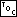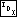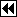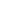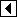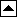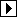Here's the code for the symmetric case, where the deleted node was in the right subtree of its parent.

```177. <Update y's balance factor after right-side AVL deletion 177> =
y->avl_balance–;
if (y->avl_balance == -1)
break;
else if (y->avl_balance == -2)   {
struct avl_node *x = y->avl_link;
if (x->avl_balance == +1)       {
struct avl_node *w;
<Rotate left at x then right at y in AVL tree 156>
pa[k - 1]->avl_link[da[k - 1]] = w;
}     else       {